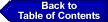### Benchmarks for Science Literacy: Chapter 15THE RESEARCH BASE

9 THE MATHEMATICAL WORLD

9B) SYMBOLIC RELATIONSHIPS

Research on Symbolic Relationships examines student understanding of the concepts of variable and algebraic equality, their ability to construct and interpret graphs, and their ability to solve algebraic equations. Several reviews on the literature base in this area are available (Herscovics, 1989; Kieran, 1989, 1992; Leinhardt et al., 1990).

Variables. Students have difficulty understanding how symbols are used in algebra (Kieran, 1992). They are often unaware of the arbitrariness of the letters chosen to represent variables in equations (Wagner, 1981). Middle-school and high-school students may regard the letters as shorthand for single objects, or as specific but unknown numbers, or as generalized numbers before they understand them as representations of variables (Kieran, 1992). These difficulties tend to persist even after instruction in algebra (Carpenter et al., 1981) and are evident even in college students (Clement, 1982). Long-term experience (3 years) in elementary computer programming has been shown to help middle-school students overcome these difficulties, although short-term experiences (less than 6 months) are less successful (Kieran, 1992; Sutherland, 1987).

Graphs. Students of all ages often interpret graphs of situations as literal pictures rather than as symbolic representations of the situations (Leinhardt, Zaslavsky, & Stein, 1990; McDermott, Rosenquist, & van Zee, 1987). Many students interpret distance/time graphs as the paths of actual journeys (Kerslake, 1981). In addition, students confound the slope of a graph with the maximum or the minimum value and do not know that the slope of a graph is a measure of rate (McDermott et al., 1987; Clement, 1989). When constructing graphs, middle-school and high-school students have difficulties with the notions of interval scale and coordinates even after traditional instruction in algebra (Kerslake, 1981; Leinhardt et al., 1990; Vergnaud & Errecalde, 1980; Wavering, 1985). For example, some students think it is legitimate to construct different scales for the positive and the negative parts of the axes. Alternatively, students think that the scales on the X and Y axes must be identical, even if that obscures the relationship. When interpreting graphs, middle-school students do not understand the effect that a scale change would have on the appearance of the graph (Kerslake, 1981). Finally, students read graphs point-by-point and ignore their global features. This has been attributed to algebra lessons where students are given questions that they could easily answer from a table of ordered pairs. They are rarely asked questions about maximum and minimum values; intervals over which a function increases, decreases or levels off; or rates of change (Herscovics, 1989).

Students have difficulty translating between graphical and algebraic representations, especially moving from a graph into an equation (Leinhardt et al., 1990). Results from the second study of the National Assessment for Educational Progress showed, for instance, that given a line with indicated intercepts, only 5% of 17-year-olds could generate the equation (Carpenter et al., 1981).

Little is known about how graphic skills are learned and how graph production is related to graph interpretation. Microcomputer-based Laboratories (MBLs) are known to improve the development of students' abilities to interpret graphs. For instance, MBLs can help middle-school students learn that a graph is not a picture and overcome the height/slope confusion mentioned above (Mokros & Tinker, 1987).

Algebraic equations. Students of all ages often do not view the equality sign of equations as a symbol of the equivalence between the left and the right side of the equation, but rather interpret it as a sign to begin calculating (Kieran, 1992). For example, middle-school students may not accept statements like 3x + 4 = x + 8 as legitimate because they think the right side should indicate the answer. Introducing the equal sign from the beginning as a symbol indicating "equivalence" between arithmetic equalities can ameliorate this difficulty (Kieran, 1981).

Beginning algebra students use various intuitive methods for solving algebraic equations (Kieran, 1992). Some of these methods may help their understanding of equations and equation solving. Students who are encouraged initially to use trial-and-error substitution develop a better notion of the equivalence of the two sides of the equation and are more successful in applying more formal methods later on (Kieran, 1988). By contrast, students who are taught to solve equations only by formal methods may not understand what they are doing. Students who are taught to use the method of "transposing" are found to only mechanically apply the change side/change sign rule (Kieran, 1988, 1989).

Students of all ages can often solve algebraic equations without a deeper understanding of what a solution is. For example, middle- and high-school students do not realize that an incorrect solution, when substituted into the equation, will yield different values for the two sides of the equation (Greeno, 1982; Kieran, 1984). More research is needed to identify how students can come to understand what a solution means and why anyone would want to find it.